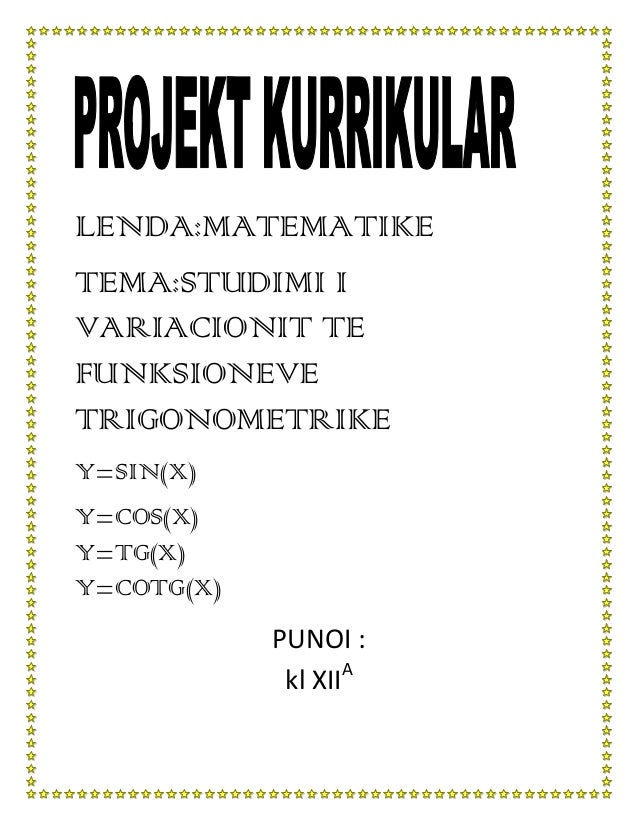Successfully reported this slideshow.
We use your LinkedIn profile and activity data to personalize ads and to show you more relevant ads. You can change your ad preferences anytime.Upcoming SlideShare
×

# Projekti i matematikes

35,970 views

Published on

ky projekt i matematikes eshte punuar nga nje nxenese e shkolles se mesme te pergjithshme manez

• Full Name
Comment goes here.

Are you sure you want to Yes No
Your message goes here• Be the first to comment

### Projekti i matematikes

1. 1. LENDA:MATEMATIKETEMA:STUDIMI IVARIACIONIT TEFUNKSIONEVETRIGONOMETRIKEY=SIN(X)Y=COS(X)Y=TG(X)Y=COTG(X) PUNOI : A kl XII
2. 2. Ne fillim shqyrtojme variacionin e funksionit y=sinx 1. Bashkesia e percaktimit per kete funksion eshte x€R(Sipas perkufizimeve,cdo vlere reale te x -it I lidhim nje vlere te vetme te sinx dhe nje vlere te vetme te cosx .Prandaj funksionet y=sinx dhe y=cosx kane bashkesi percaktimi R) 2. Shqyrtojme ciftesine e funksionit dhe shohim nese funksioni eshte periodik f(-x)≠f(x) sinx=>jo funksion cift f(-x)=-f(x)=>si funksioni eshte periodik (x+T)=f(x) vetem kur T=2π 3. Njehsojme derivatin dhe studiojme shenjen e tij y’=(sinx)’=cosx =>cosx=o d.m.th ne dhe ne 0 π 2π x f(x)’ f(x) max minFunksioni sinx eshte rrites ne ]0, ]u[ ,2π*Funksioni eshte zbrites ne [ f (max)=f( ) =sin =1 A( ,1) f(min)=f( )=sin =-1 B( ,-1)
3. 3. 4. Gjejme derivatin e dyte dhe studiojme shenjen e tij y’’=(cosx )’’ =-sinx =>-sinx=o d.m.th ne 0 , π dhe 2π x 0 π 2π f(x)’’ f(x) p.inf p,inf p.inf Grafiku I funksionit f(x) eshte I myset ne [0, [u] ,π]Dhe I luget ne [π. pikat x=0 ;x=π;x=2π jane pika infleksioni.Gjejmeordinatat e tyre jane per f(0)=sin0=o ; per f(π)=sinπ=0 ;per f(2π)=sin 2π=0c=(0,0);d(π,0);e(2π,0) 5. Gjejme limitet e funksionit kur ; ; =-1 6. Grafiku I ketij funksioni nuk ka asimptote vertikale meqenese ehte I percaktuar ne R,dhe as asimptote horizontale pasi limx->+∞=+∞, dhe 7. Gjejme pikat ku grafiku prêt boshtin => =>G(0;0) =>sinx =o per x=0 , x=π, x=2π Grafiku e pret boshtin ne pikat(0,0)dhe ne pikat (0,0);(π,0)(2π,0)
4. 4. 8. Bejme tabelen permbledhese 0 π 2π x f(x)’’ f(x)’ f(x)+ 9. Ndertojme grafikun e funksioneve A ( ,1); B ( ,1);C(0,0) D(π,0)
5. 5. Studiojme variacionin e funksionit y=cosx 1. B .percaktimit xeR 2. f(-x) pra eshte funksiom cift f(-x)=f(x)=>cos(-x)=cosx=>funksioni eshte cift f(x+T)=f(x)=>funksioni eshte periodic vlen kur perioda T=2π 3. f(x)’=cosx’=-sinx -sinx=0 per x=0,x=π x=2π x 0 π 2π f(x)’ f(x)Funksioni f(x)eshte rrites ne [π; [u] ;2π+Zbrites ne [0; [u] π]f(0)=cos0=1f(π)=cosπ=-1f(2π)=cos2π=1A(0,1);B(π,-1);C(2π,1)
6. 6. 4. Njehsojme derivatin e dyte f(x)’’=-sinx’=-cosx -cosx=0 per x= x 0 π 2π f(x)’’ - + + - f(x) p.infl p.inflfunksioni fx eshte I myset ne ]0, ]u[ ,2π[funksioni fx eshte I luget ne [ ,π[u]π, ]f( )=cos =of( )=cos =0 D( o)E ( ,o) 5. 6. Meqe ky funksion eshte I percaktuar ne R nuk ka A.V Dhe meqenese 7. Gjejme pikat ku prêt boshtet kordinative =>per x= dhe x= =>( ,0) ( ,0) =>cos0=1=>(0,1)
7. 7. 8. Bejme tabelen permbledhese x 0 π 2π f(x)’ - - + + f(x)’’ - + + - f(x)9. Ndertojme grafikunStudiojme variacionin e tgx1. B.p cosx x2. f(- x)
8. 8. )= f(x)nuk eshte tek f(x+T)=f(x)=>T=π ky funksion eshte periodic3. ( )’= .>0 prandaj ky funksion eshte rrites Duke qene se b.p eshte cosx≠odhe x e] [ - + + + f(x)’ f(x)4. - 0f(x)’’ - +f(x)
9. 9. Funksioni fx eshte I myset ne]- ,o[I luget ne ]o [Per f(0)p.infleksioniF(0) A(0,0) 5. 6. Asimptota vertikale eshte cosx=0,x= A.h ska pasi 7. Pikeprerjet =>dhe kemi(0,0) 8. Ndertojme tabelen permbledhese - 0 f(x)’ + + f(x)’’ - + f(x)
10. 10. 9. Ndertojme grafikunStudiojme variacionin e funksionit y=cotg x 1. Bashkesia e percaktimit sin(x 2. Studiojme ciftesine e funksionit dhe shohim nese funksioni eshte periodic 3. Njehsojme derivatin e pare
11. 11. ⁻π πf(x)’ - ------f(x)4. Gjejme derivatin e dyte Pra kemi pikat( ;0)
12. 12. π f(x)’’ + - f(x) p.in funksioni f(x)eshte I myset ne =0 A(5. Gjejme limitine funksionit6. Gjejme A.Vertikale cotgx= ;x=0 ;x=π A.horizontale nuk ka pasiBejme tabelen permbledhese
13. 13. πf(x)’ - -f(x)’’ - + 7. Ndertojme grafikun y x